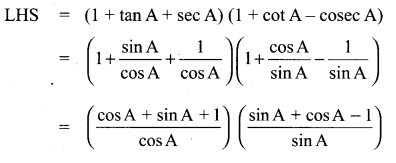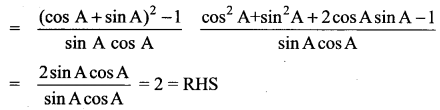## Tamilnadu Samacheer Kalvi 11th Maths Solutions Chapter 3 Trigonometry Ex 3.1

11th Maths Exercise 3.1 Answers Question 1.
Identify the quadrant in which an angle of each given measure lies
(i) 25°
(ii) 825°
(iii) -55°
(iv) 328°
Solution:
(ii) 825° = 105° (90° + 15°) = II quadrant
(iv) 328° = IV quadrant (270° + 58°)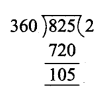(v) -230° = 360° – 230° = 130° = (90° + 40°) II quadrant

11th Maths Exercise 3.1 Question 2.
For each given angle, find a coterminal angle with measure of θ such that θ° < θ < 360°
(i) 395°
(ii) 525°
(iii) 1150°
(iv) -270°
(v) -450°
Solution:
(i) 395° = 360° + 35°
∴ coterminal angle = 35°

(ii) 525° – 360°= 165°
coterminal angle = 165°

(iii) 1150° = 360 × 3 + 70° = 70°
coterminal angle = 70°

(iv) -270° = coterminal angle =+90° {270° + 90° = 360°}

(v) -450° = -360° – 90° = -90°
∴ coterminal angle = 360° – 90° = 270°

Exercise 3.1 Class 11 Maths Solutions State Board Question 3.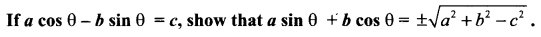Solution:
a cos θ – b sin θ = c
⇒ (a cos θ – b sin θ)2 = c2
(i.e) a2 cos2 θ + b2 sin2 θ – 2ab sin θ cos θ = c2
(i.e) a2 (1 – sin2 θ) + b2 (1 – cos2 θ) – 2ab sin θ cos θ = c2
a2 – a2 sin2 θ + b2 – b2 cos2 θ – 2ab sin θ cos θ = c2
a2 + b2 – c2 = a2 sin2 θ + b2 cos2 θ + 2ab sin θ cos θ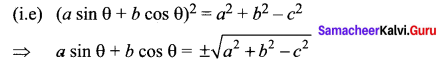Exercise 3.1 Class 11 Maths State Board Question 4.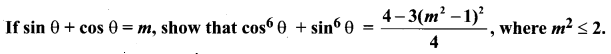Solution: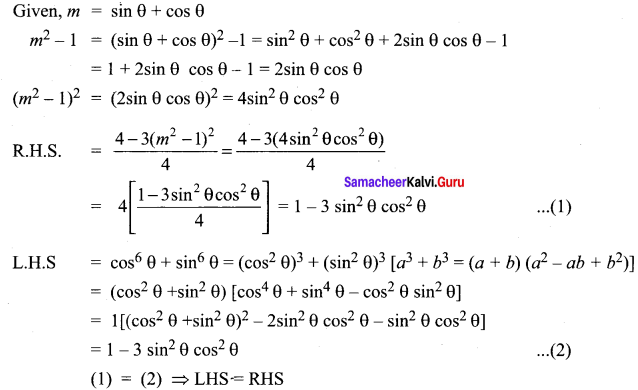Samacheer 11th Maths Solution Question 5.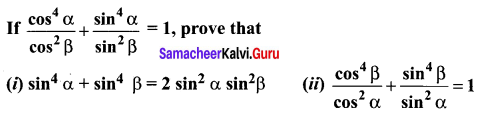Solution: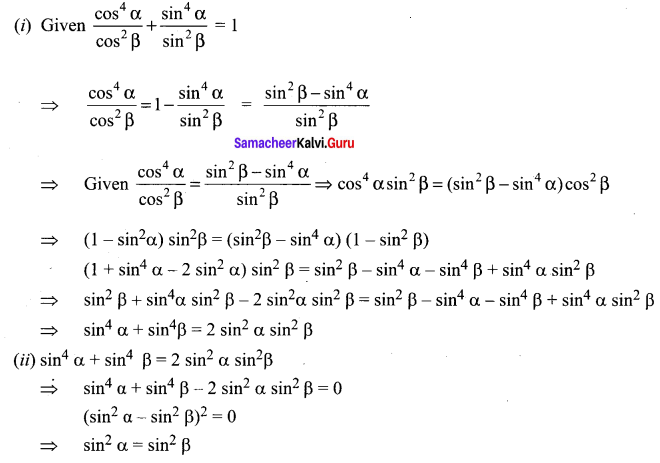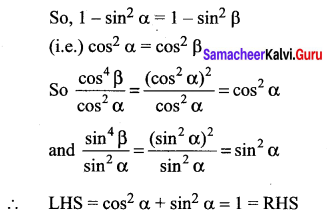Samacheer Kalvi 11 Maths Solutions Question 6.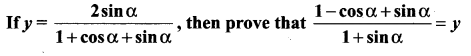Solution: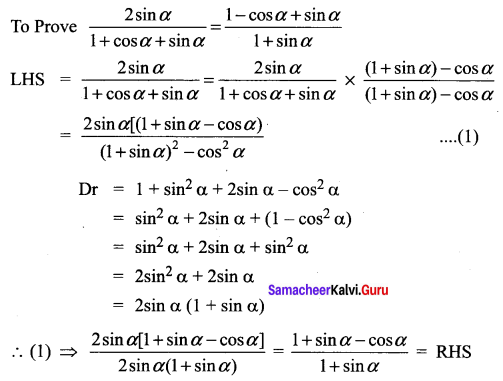Samacheer Kalvi 11th Maths Guide Question 7.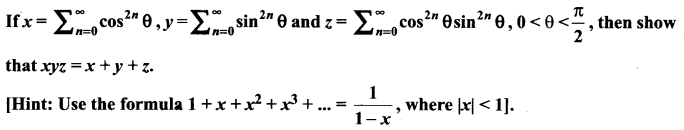Solution: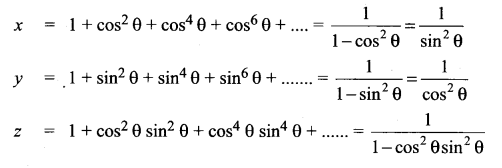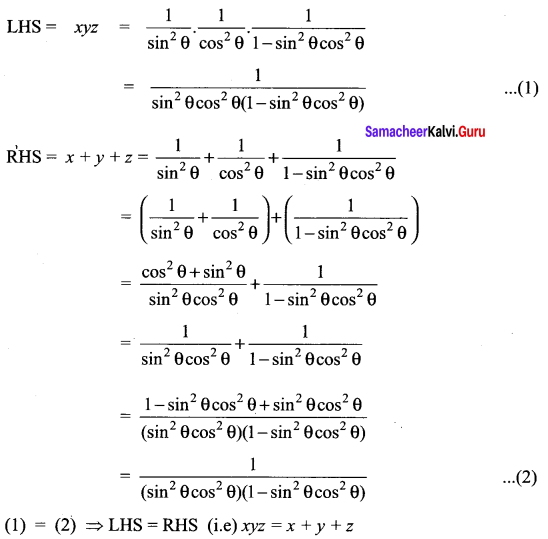Samacheer Kalvi 11 Maths Question 8.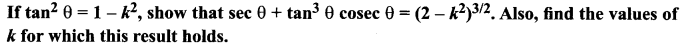Solution: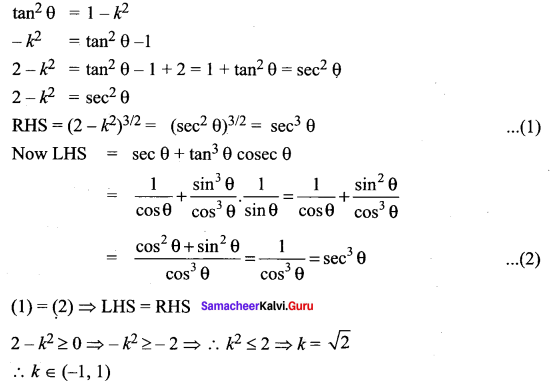Samacheer Kalvi 11th Maths Example Sums Question 9.
If sec θ + tan θ = p, obtain the values of sec θ, tan θ and sin θ in terms of p.
Solution:
Given, sec θ + tan θ = p
we know sec2 θ – tan2 θ = 1
(i.e) (sec θ + tan θ) (sec θ – tan θ) = 1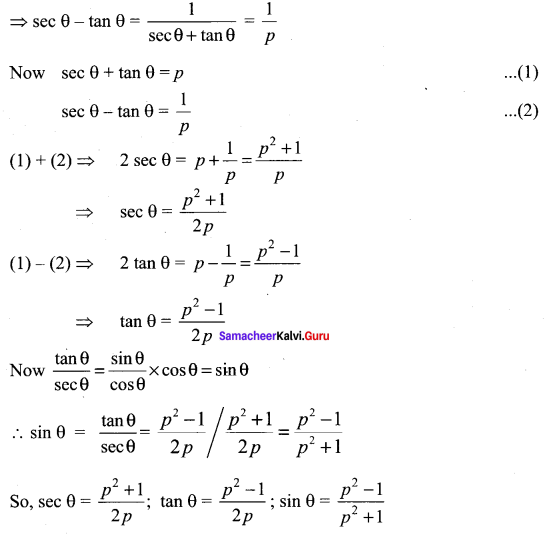Samacheer Kalvi Class 11 Maths Solutions Question 10.
If cot θ (1 + sin θ) = 4m and cot θ (1 – sin θ) = 4n, then prove that (m2 – n2)2 = mn.
Solution:
cot θ (1 + sin θ) = 4m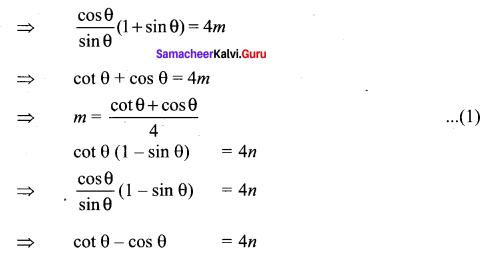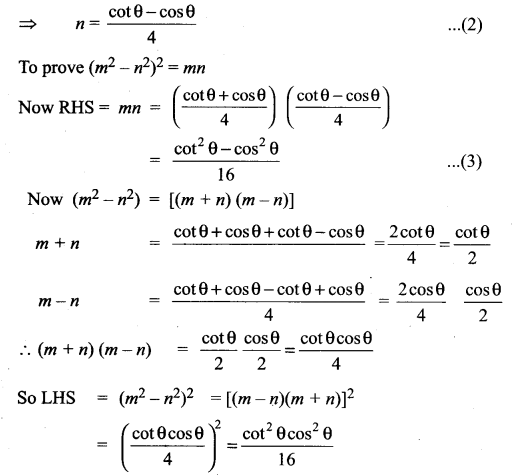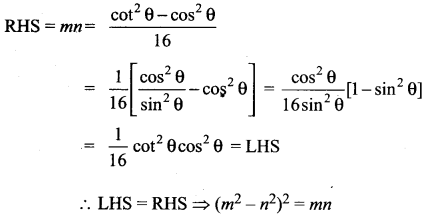Samacheer Kalvi Class 11 Maths Question 11.
If cosec θ – sin θ = a3 and sec θ – cos θ = b3, then prove that a2b2 (a2 + b2) = 1.
Solution: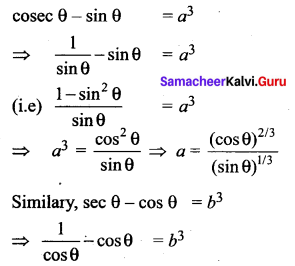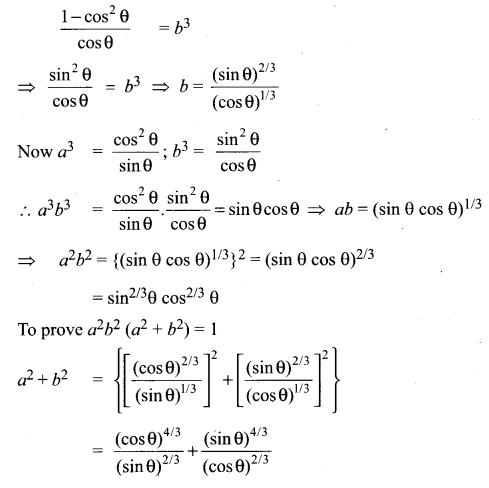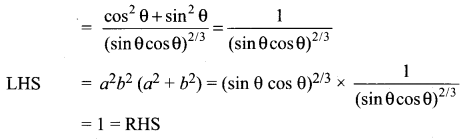Samacheer Class 11 Maths Solutions Question 12.
Eliminate θ from the equations a sec θ – c tan θ = b and b sec θ + d tan θ = c.
Solution:
Taking sec θ = X and tan θ = Y we get the equations as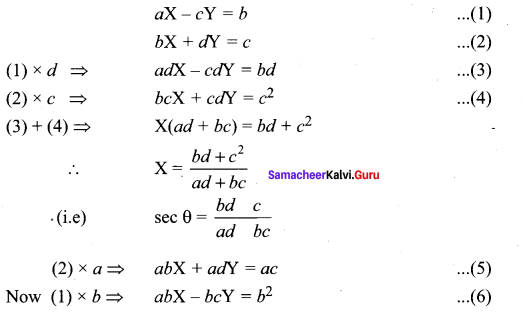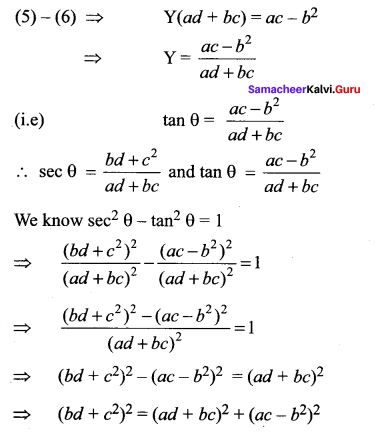### Samacheer Kalvi 11th Maths Solutions Chapter 3 Trigonometry Ex 3.1 Additional Equations

11th Maths 3rd Chapter Solutions Question 1.
Prove that (sec θ + cos θ) (sec θ – cos θ) = tan2 θ + sin2 θ
Solution:
(sec θ + cos θ) (sec θ – cos θ) = sec2 θ – cos2 θ
= (1 + tan2 θ ) – (1 – sin2 θ)
= tan2 θ + sin2 θ = RHS

Class 11 Maths Solutions Samacheer Kalvi Question 2.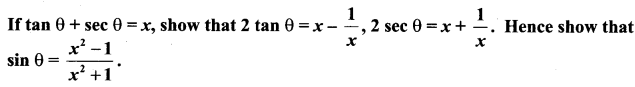Solution: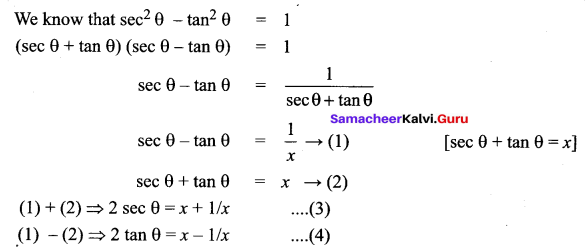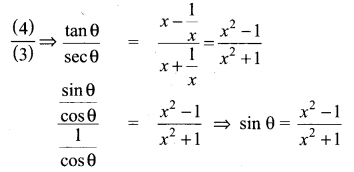11th Samacheer Maths Solutions Question 3.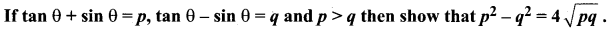Solution: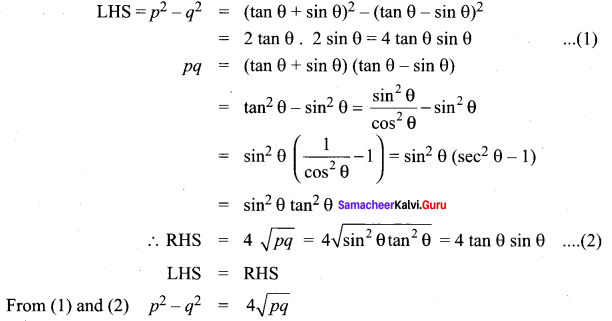Samacheer Kalvi Guru 11th Maths Question 4.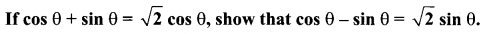Solution: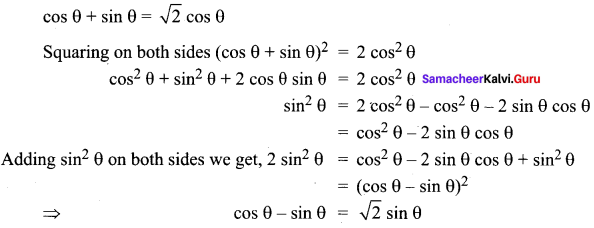Samacheer Kalvi 11th Maths Question 5.
Prove that (1 + tan A + sec A) (1 + cot A – cosec A) = 2
Solution:
LHS = (1 + tan A + sec A) (1 + cot A – cosec A)